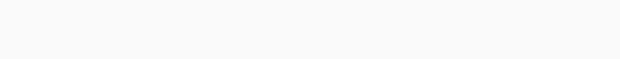#Reasoning Questions – Machine Input-Output(New Pattern) Set 37

Hello Aspirants.

Welcome to Online Reasoning Section with explanation in AffairsCloud.com. Here we are creating Best question samples from Machine Input-output, which is common for all the IBPS, SBI , LIC, and other competitive exams. We have included questions that are repeatedly asked in bank exams !!!

(Directions 1 – 5): Study the given information carefully and answer the given questions:
An input-output is given in different steps. Some mathematical operations are done in each step. No mathematical operation is repeated in next step.1. What is the multiplication of all the numbers of step 2?
1) 8
2) 6
3) 12
4) 15
5) None of these
Solution:
In first step:
Multiply 1st and 2nd number of box 1 and 4 respectively: 5 × 4 = 20
Multiply 2nd and 1st number of box 1 and 4 respectively: 3 × 2 = 6
Multiply 1st and 2nd number of box 2 and 5 respectively: 7 × 3 = 21
Multiply 2nd and 1st number of box 2 and 5 respectively: 2 × 4 = 8
Multiply 1st and 2nd number of box 3 and 6 respectively: 6 × 7 = 42
Multiply 2nd and 1st number of box 3 and 6 respectively: 2 × 3 = 6In second step:
Add 1st and 3rd number of box 1 and subtract it from 2nd number of box 1 = 6 + 2 = 8, 8 – 0 = 8
Add 1st and 3rd number of box 2 and subtract it from 2nd number of box 2 = 8 + 2 = 10, 10 – 1 = 9
Add 1st and 3rd number of box 3 and subtract it from 2nd number of box 3 = 6 + 4 = 10, 10 – 2 = 8

In third step:
Divide 2nd number from 1st number = 9 ÷ 8 = 1.12
Divide 3rd number from 2nd number = 8 ÷ 9 = 0.89

In fourth step:
Subtract both the numbers = 1.12 – 0.89 = 0.23In first step:
Multiply 1st and 2nd number of box 1 and 4 respectively: 6 × 4 = 24
Multiply 2nd and 1st number of box 1 and 4 respectively: 4 × 1 = 4
Multiply 1st and 2nd number of box 2 and 5 respectively: 4 × 7 = 28
Multiply 2nd and 1st number of box 2 and 5 respectively: 3 × 3 = 9
Multiply 1st and 2nd number of box 3 and 6 respectively: 3 × 6 = 18
Multiply 2nd and 1st number of box 3 and 6 respectively: 8 × 1 = 8

In second step:
Add 1st and 3rd number of box 1 and subtract it from 2nd number of box 1 = 4 + 2 = 6, 6 – 4 = 2
Add 1st and 3rd number of box 2 and subtract it from 2nd number of box 2 = 9 + 2 = 11, 11 – 1 = 3
Add 1st and 3rd number of box 3 and subtract it from 2nd number of box 3 = 8 + 1 = 9, 9 – 8 = 1

In third step:
Divide 2nd number from 1st number = 3 ÷ 2 = 1.5
Divide 3rd number from 2nd number = 1 ÷ 3 = 0.33

In fourth step:
Subtract both the numbers = 1.5 – 0.33 = 1.17

2. Which is the largest number obtained in step 1?
1) 188
2) 244
3) 249
4) 289
5) 250

3. If the third number of all the boxes is halved and then added the half numbers, what is the result in step 1?
1) 10.5
2) 12.5
3) 8.5
4) 11.5
5) None of these

4. What is the sum of numbers in step 3?
1) 0.66
2) 0.74
3) 1.83
4) 1.66
5) 1.35

5. Which is the following number obtained in last step?
1) 1.07
2) 1.77
3) 1.67
4) 1.17
5) None of these

Directions (6 – 10): Study the given information carefully and answer the given questions:
An input-output is given in different steps. Some mathematical operations are done in each step. No mathematical operation is repeated in next step.1. Find the addition of two numbers obtained in step III?
1) 1.5
2) 3
3) 7
4) 3.5
5) None of these
Solution:
In first step:
5 × 1 = 5, 3 × 2 = 6
1 × 3 = 3, 2 × 4 = 8
2 × 4 = 8, 2 × 4 = 8In second step:
Adding all the first digits: 5 + 3 + 8 = 16
Adding all the second digits: 6 + 8 + 8 = 22

In third step:
1 + 6 = 7, 7/2 = 3.5
2 + 2 = 4, 4/2 = 2

In fourth step:
3.5 – 2 = 1.52. Find the sum of numbers obtained in 1st step?
1) 232
2) 185
3) 188
4) 183.5
5) None of these

3. What is the multiplication of the numbers in step II?
1) 442
2) 452
3) 472
4) 462
5) 422

4. Which of the following is the lowest number in step I?
1) 86
2) 57
3) 80
4) 89
5) None of these